SPM Practice (Long Questions)

Question 9 (12 marks):
(a) Diagram 7.1 shows K (5, 1) drawn on a Cartesian plane.Diagram  7.1

Transformation T is a translation
Transformation P is a reflection in the line y = 2.
State the coordinates of the images of point K under each of the following transformations:
(i) T2,
(ii) TP.

(b) Diagram 7.2 shows two pentagons KLMNP and QRSTU, drawn on a Cartesian plane.Diagram  7.2

(i)
Pentagon QRSTU  is the image of pentagon KLMNP under the combined transformation WV.
Describe in full the transformation:
(a) V,
(b) W.

(ii)
It is given that pentagon QRSTU represents a region with an area of 90 m2.
Calculate the area, in m2, of pentagon KLMNP.

Solution:
(a)(i)
TT = K(5, 1) → T1 → K’(2, 5) ) → T2 → K’’(–1, 9)
(ii) TP =  K(5, 1) → P → K’(5, 3) → T → K’’(2, 7)

(b)(b)(i)(a)

V: 90o rotation in clockwise direction at point K(3, 7).

(b)(i)(b)
W: Enlargement at point (1, 6) with scale factor 3.

(b)(ii)
Area of QRSTU = (scale factor)2 × area of object
Area of QRSTU = 32 × Area of KLMNP
90 m2 = 9 × Area of KLMNP
Area of KLMNP = 90/9 = 10 m2

6.3 SPM Practice (Long Questions)

Question 12 (5 marks):
Diagram 7 shows a distance-time graph for the journey of a car from Kuala Lumpur to Ipoh.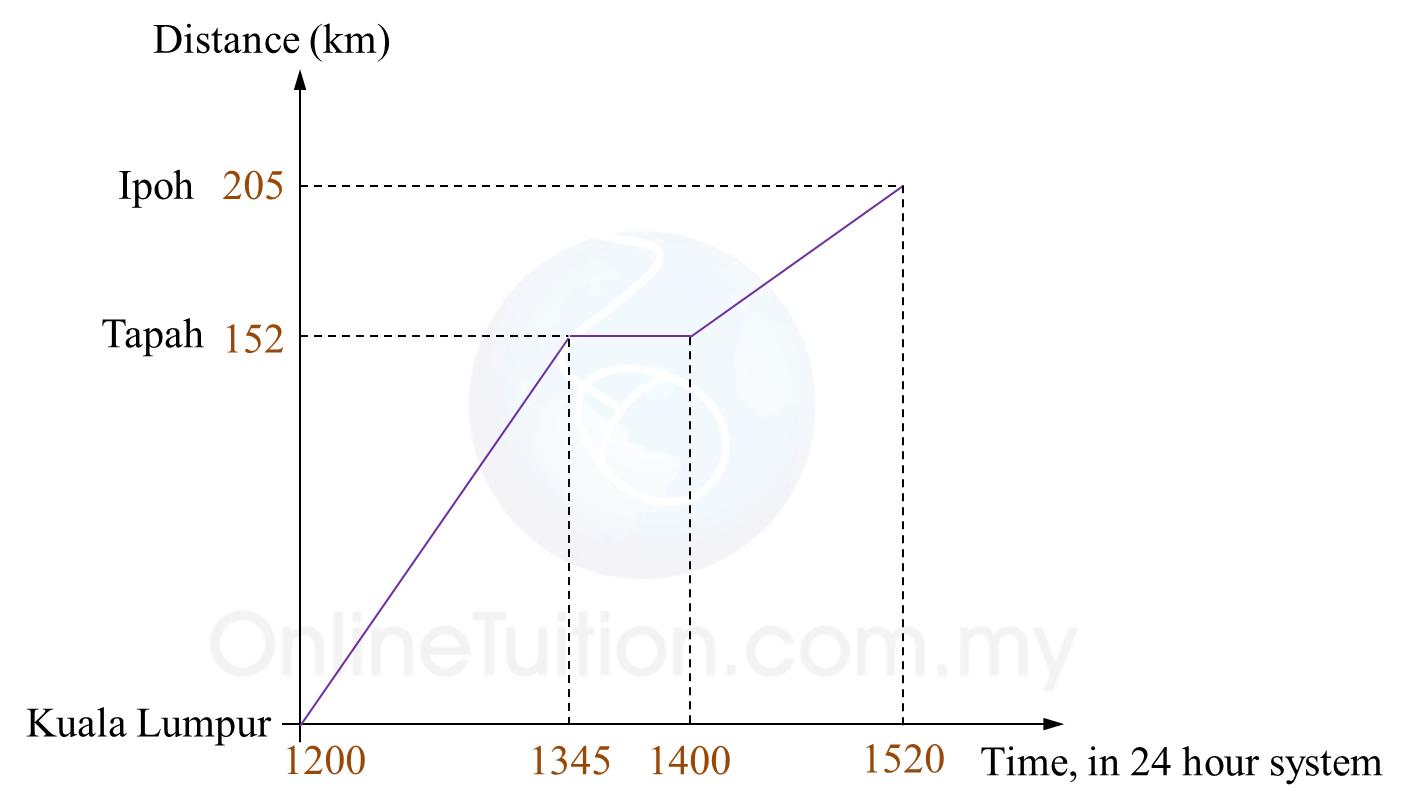(a) State the duration of time, in minutes, the driver stopped and rest at Tapah.
(b) Calculate the speed, in kmh-1, of the car from Kuala Lumpur to Tapah.
(c) Calculate the average speed, in kmh-1, of the car for whole journey.

Solution:
(a)
1400 – 1345 = 15 minutes
The driver stopped and rest at Tapah for 15 minutes.

(b)

(c)

6.3 SPM Practice (Long Questions)

Question 11 (6 marks):
Diagram 6 shows the distance-time graph of Ursula, Janet and Maria in a 100 m race.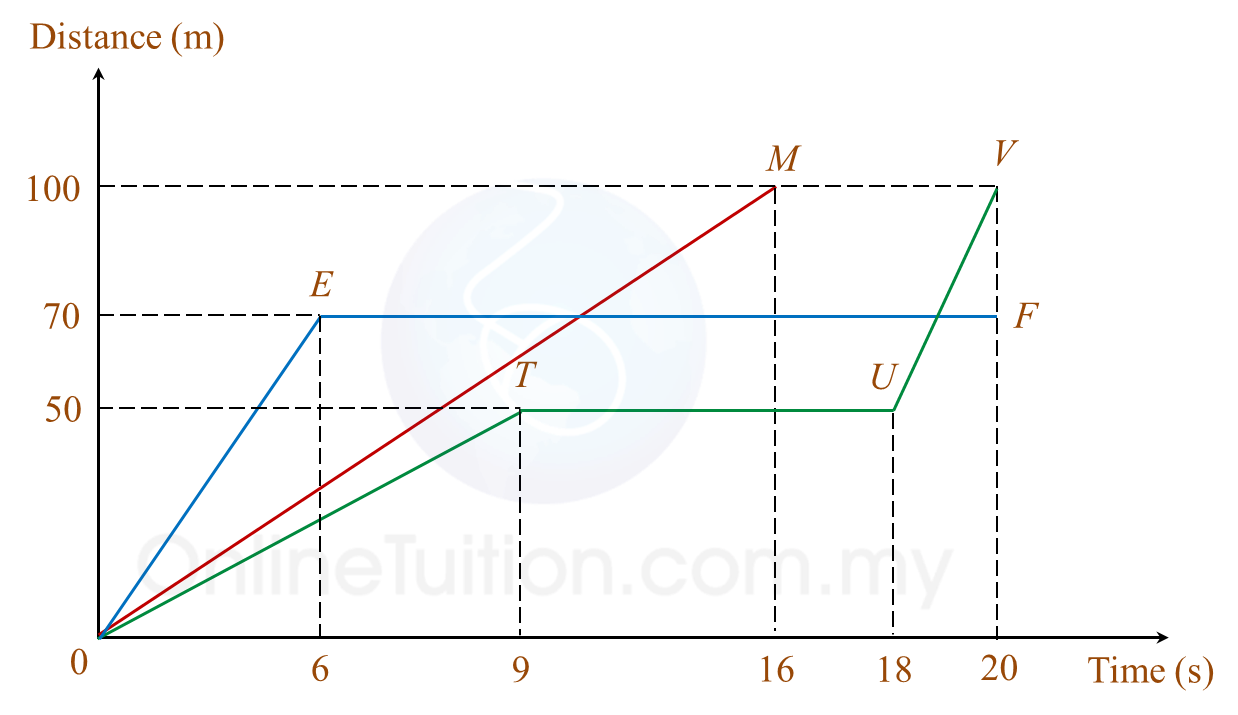Diagram 6(a) Who won the race?

(b)
During the race, Ursula slipped and fell over. After that, she continued her run.
State the duration, in seconds, before Ursula continued her run.

(c)
During the race, Janet was injured and she stopped running.
State Janet's distance, in m, from the finishing line when she stopped running.

(d)
Calculate the average speed, in ms-1, of Ursula.

Solution:
(a)
Maria won the race: 100 km in 16 seconds.

(b)
Duration before Ursula continued her run from slipping and falling over
= 18 seconds – 9 seconds
= 9 seconds

(c)
Janet's distance from the finishing line when she stopped running
= 100 m – 70 m
= 30 m

(d)

7.5 SPM Practice (Long Questions)

Question 10 (5 marks):
The table below shows some members of Red Crescent Society and St John Ambulance Society that are instructed to do an outdoor tasks at various places during the Flag Day.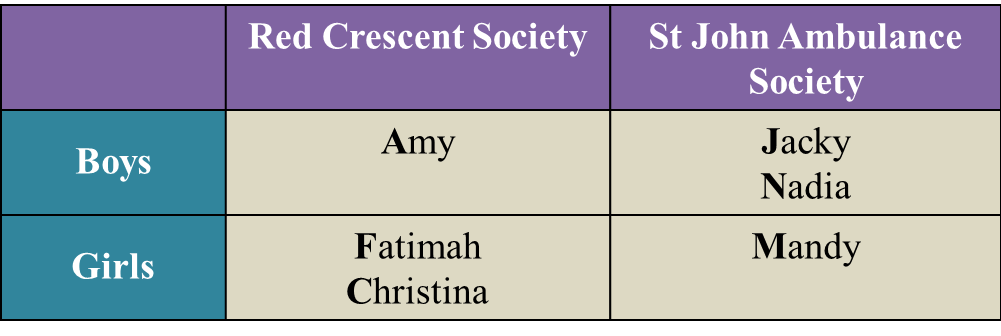Two members from the societies were dropped off random at those places.
(a) List all the possible outcomes of the event in this sample space.
You also may use the letters such as A for Amy and so on.

(b) By listing down all the possible outcomes of the event, find the probability that
(i) a boy and a girl were dropped off at a certain place.
(ii) both members that were dropped off at a certain place are from the same society.

Solution:
(a)
S = {(A, J), (A, N), (A, F), (A, C), (A, M), (J, A), (J, N), (J, F), (J, C), (J, M), (N, A), (N, J), (N, F), (N, C), (N, M), (F, A), (F, J), (F, N), (F, C), (F, M), (C, A), (C, J), (C, N), (C, F), (C, M),(M, A), (M, J), (M, N), (M, F), (M, C)}

(b)(i)
{(A, F), (A, C), (A, M), (J, F), (J, C), (J, M), (N, F), (N, C), (N, M), (F, A), (F, J), (F, N), (C, A), (C, J), (C, N), (M, A), (M, J), (M, N)}
$\begin{array}{l}\text{Probability}\\ =\frac{18}{30}\\ =\frac{3}{5}\end{array}$

(b)(ii)
{(A, F), (A, C), (F, A), (F, C), (C, A), (C, F), (J, N), (J, M), (N, J), (N, M), (M, J), (M, N)}
$\begin{array}{l}\text{Probability}\\ =\frac{12}{30}\\ =\frac{2}{5}\end{array}$

7.5 SPM Practice (Long Questions)

Question 9 (6 marks):
Diagram 10.1 shows a disc with four equal sectors and a fixed pointer. Each sector is labelled with water heater, oven, television and iron respectively. Diagram 10.2 shows a box which contains three cash vouchers, RM10, RM20 and RM50.A lucky customer in a supermarket is given a chance to spin the disc once and then draw a cash voucher from the box.
(a) List the sample space for the combination of prizes that can be won.

(b)
By listing down all the possible outcomes of the event, find the probability that

(i)
the customer wins a television or cash voucher worth RM50,

(ii)
the customer does not wins the water heater and the cash voucher worth RM20.

Solution:
(a)
S = {(Water heater, RM10), (Water heater, RM20), (Water heater, RM50), (Oven, RM10), (Oven, RM20), (Oven, RM50), (Television, RM10), (Television, RM20), (Television, RM50), (Iron, RM10), (Iron, RM20), (Iron, RM50)}

(b)(i)
{( Water heater, RM50), (Oven, RM50), (Iron, RM50), (Television, RM10), (Television, RM20), (Television, RM50)}
$\begin{array}{l}\text{Probability}\\ =\frac{6}{12}\\ =\frac{1}{2}\end{array}$

(b)(ii)

3.4 SPM Practice (Long Questions)

Question 9 (3 marks):
The Venn diagram in the answer space shows set P, set Q and set R such that the universal set ξ = P U Q U R.
(a) P’,
(b) (PQ) U R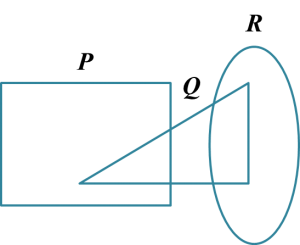Solution:
(a)(b)Question 10 (3 marks):
(a) It is given that set E = {perfect square numbers} and set F = {9, 16, 25}.
Complete the Venn diagram in the answer space to show the relationship between set E and set F.(b)
The Venn diagram in Diagram 1 shows the sets X, set Y and set Z.
The universal set, ξ = X U Y U Z.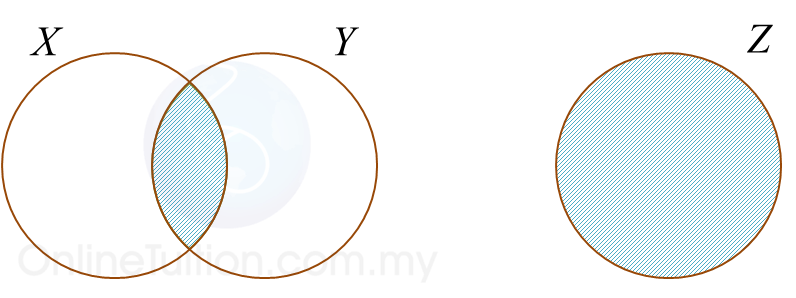Diagram 1

State the relationship represented by the shaded region between sets X, set Y and set Z.

Solution:

(a)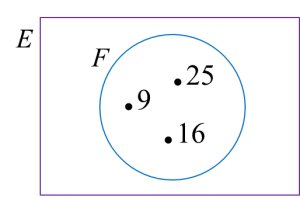(b)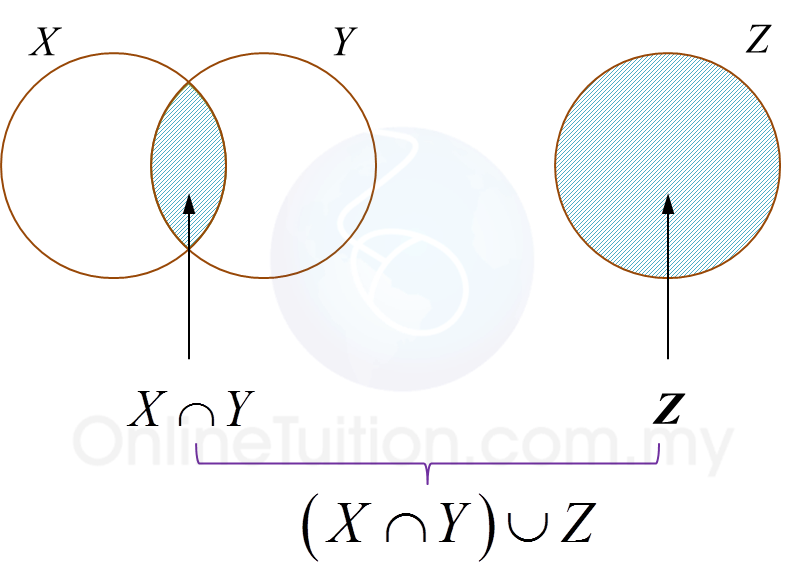Hence, relationship represented by the shaded region between sets X, set Y and set are (X ∩ Y) ∪ Z.

4.10 SPM Practice (Long Questions)

Question 11 (5 marks):
Table 8 shows the information of books purchased by Maslinda.Table 8

Maslinda purchased x history books and y Science books. The total number of books purchased is 5. The total price of the books purchased is RM17.

(a)
Write two linear equations in terms of x and y to represent the above information.

(b)
Hence, by using matrix method, calculate the value of x and of y.

Solution:
(a)
x + y = 5
4x + 3y = 17

(b)

Question 12 (6 marks):

(a) Find the value of m and of n if AB = I.
(b) Write the following simultaneous linear equation as matrix equation:
4x – 2y = 3
3xy = 2
Hence, by using matrix method, calculate the value of x and of y.

Solution:
(a)

(b)

4.6 SPM Practice (Long Questions)

Question 13 (5 marks):
(a) State whether the following statements are true statement or false statement.

(b) Diagram 7 shows the first three patterns of a sequence of patterns.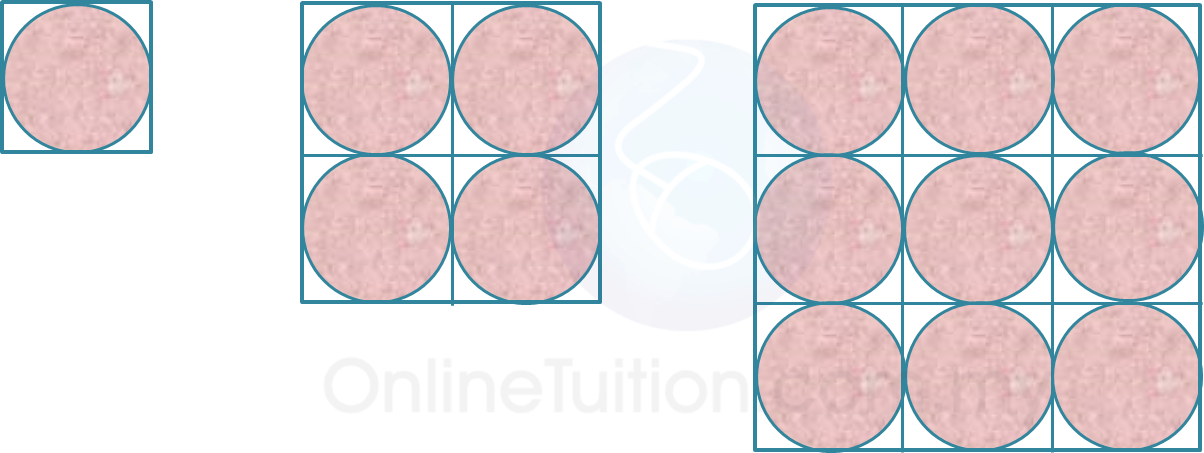Diagram 7

It is given that the diameter of each circle is 20 cm.
(i) Make a general conclusion by induction for the area of the unshaded region.

(ii)
Hence, calculate the area of the unshaded region for the 5th pattern.

Solution:
(a)(i) True

(a)(ii) False

(b)(i)
= (20 × 20) – π(10)2
= 400 – 100π
= 100 (4 – π)

= 4 × 100 (4 – π)
= 400 (4 – π)

= 9 × 100 (4 – π)
= 900 (4 – π)
100 (4 – π), 400 (4 – π), 900 (4 – π), …
102 (4 – π), 202 (4 – π), 302 (4 – π), …

General conclusion = n2 (4 – π)
n = 10, 20, 30, …

(b)(ii)

= 502 (4 – π)
= 2500 (4 – π)

4.6 SPM Practice (Long Questions)

Question 11 (5 marks):
(a) State whether the following compound statement is true or false.
2 > 3 and (–2)3 = –8

(b) Write down two implications based on the following statement:
a > b if and only if a – b > 0

(c) Table 1 shows the number of sides and the number of axes of symmetry for some regular polygons.Table 1

Make a conclusion by induction by completing the statement in the answer space:
The number of axes of symmetry for a regular polygon with n sides is __________.

Solution:
(a)

(b)
Implication 1: Jika a > b, maka a – b > 0
Implication 2: Jika a – b > 0, maka a – b > 0

(c)
The number of axes of symmetry for a regular polygon with n sides is
Number of sides of regular polygon = Number of axes of symmetry of regular polygon

Question 12 (5 marks):
(a) State whether the following statement is true or false.(b)
Write down the converse of the following implication: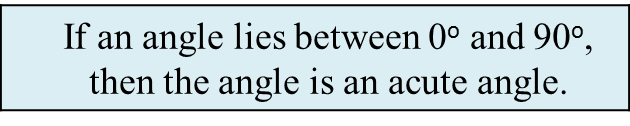(c)
Write down Premise 2 to complete the following argument:
Premise 1   :  If x is an odd number, then x is not divisible by 2.
Premise 2   : ………………………………………………………
Conclusion : 24 is not an odd number.

(d)
Based on the information below, make one conclusion by deduction for the surface area of sphere with radius 9 cm.Solution:
(a)
True

(b)
If an angle is an acute angle, then the angle lies between 0o and 90o.

(c)
24 is divisible by 2.

(d)
4π(9)2 = 324π
Surface area of the sphere is 324π.

5.7 SPM Practice (Long Questions 6)

Question 11 (5 marks):
Diagram 4 shows a parallelogram drawn on a Cartesian plane which represents the locations of Rahman's house, a cinema, a school and a shop.It is given that the scale is 1 unit : 1 km.
(a) Calculate the distance, in km, between Rahman's house and the school.
(b) Find the equation of the straight line that links the school to the cinema.

Solution:
(a)
2y = 3x + 15
When y = 0
3x + 15 = 0
3x = –15
x = –5

Rahman's house = (–5, 0)
School = (3, 0)

Distance, between Rahman's house and the school
= 3 – (– 5)
= 8 units
= 8 km

(b)

Question 12 (6 marks):
Diagram 6 shows two parallel straight lines, JK and LM, drawn on a Cartesian plane.
The straight line KM is parallel to the x-axis.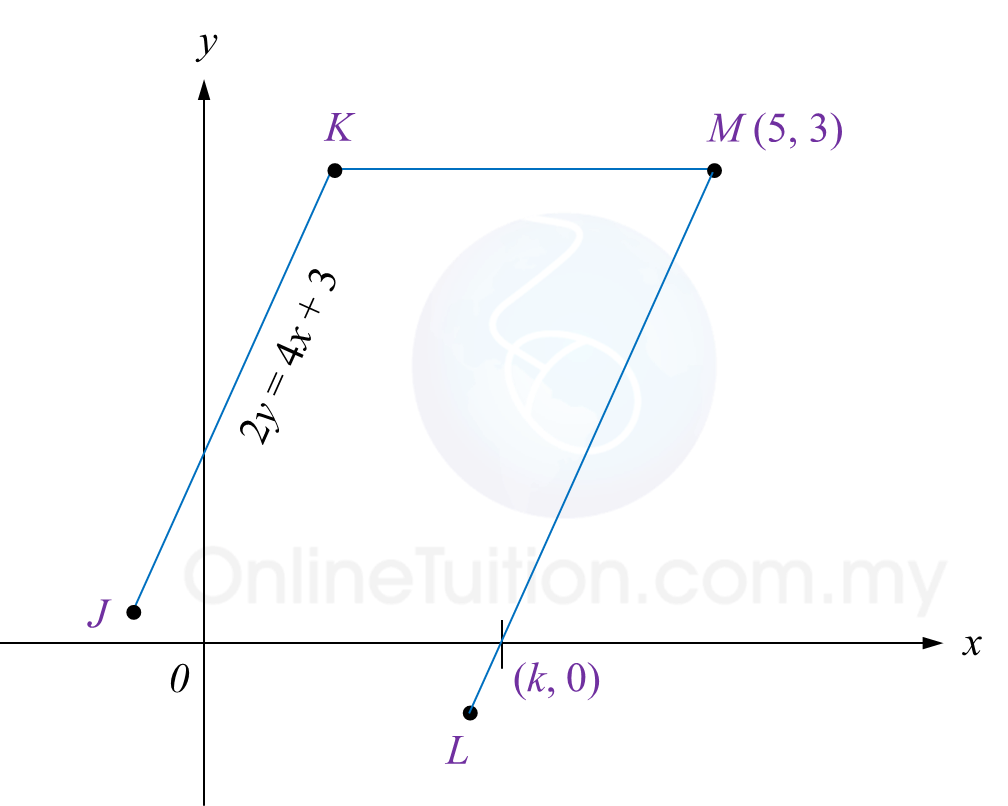Diagram 6

Find
(a) the equation of the straight line KM,
(b) the equation of the straight line LM,
(c) the value of k.

Solution:
(a)

The equation of the straight line KM is y = 3

(b)

(c)